Question

4. Two conducting spheres are far apart. The smaller sphere has radius R and carries a...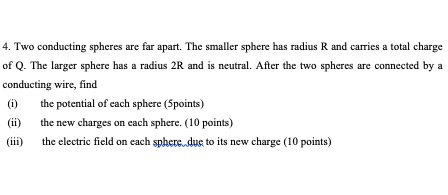4. Two conducting spheres are far apart. The smaller sphere has radius R and carries a total charge of Q. The larger sphere has a radius 2R and is neutral. After the two spheres are connected by a conducting wire, find (1) the potential of each sphere (Spoints) the new charges on each sphere. (10 points) (iii) the electric field on each sphere due to its new charge (10 points)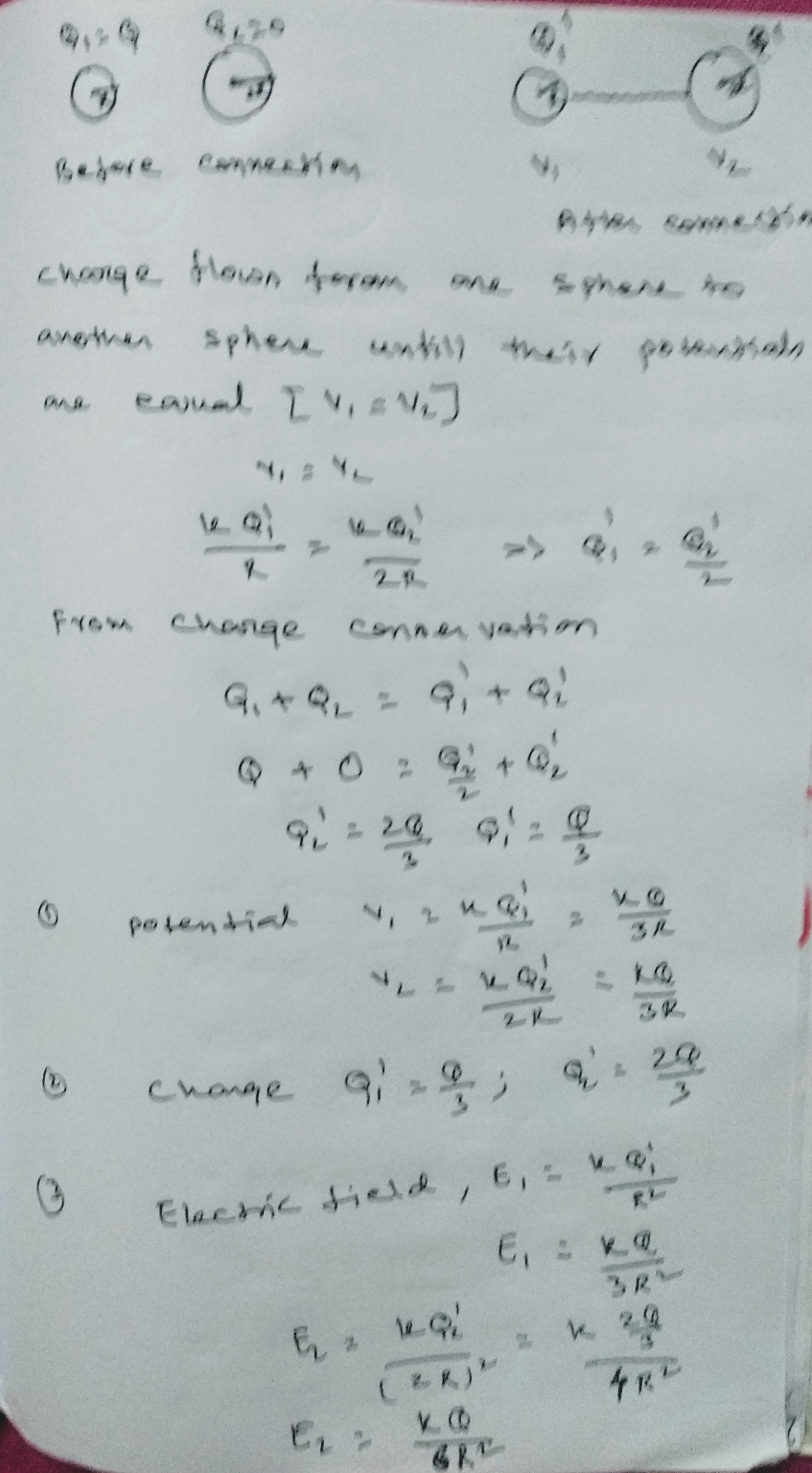Earn Coins

Coins can be redeemed for fabulous gifts.

Similar Homework Help Questions
• 4. Two conducting spheres are far apart. The smaller sphere has radius R and carries a...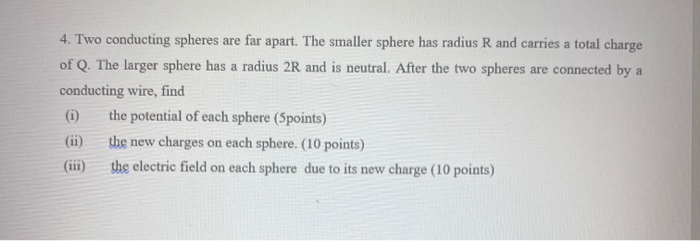4. Two conducting spheres are far apart. The smaller sphere has radius R and carries a total charge of Q. The larger sphere has a radius 2R and is neutral. After the two spheres are connected by a conducting wire, find (1) the potential of each sphere (Spoints) the new charges on each sphere. (10 points) (iii) the electric field on each sphere due to its new charge (10 points)

• 4. Two conducting spheres are far apart. The smaller sphere has radius R and carries a...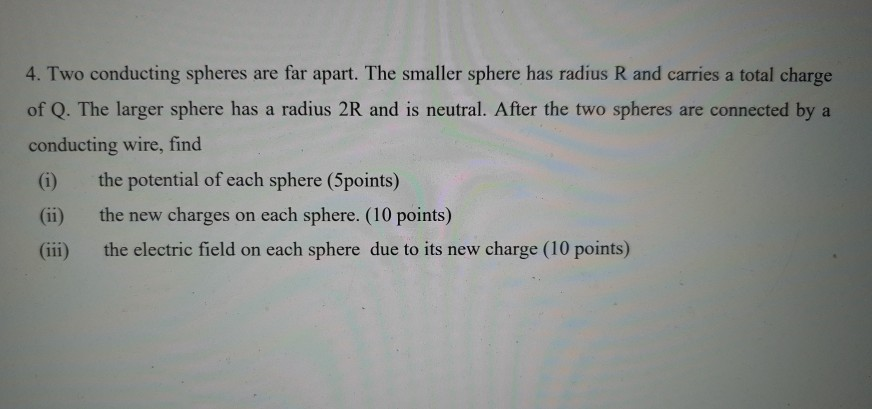4. Two conducting spheres are far apart. The smaller sphere has radius R and carries a total charge of Q. The larger sphere has a radius 2R and is neutral. After the two spheres are connected by a conducting wire, find the potential of each sphere (5points) the new charges on each sphere. (10 points) (iii) the electric field on each sphere due to its new charge (10 points)

• 4. Two conducting spheres are far apart. The smaller sphere has radius R and carries a...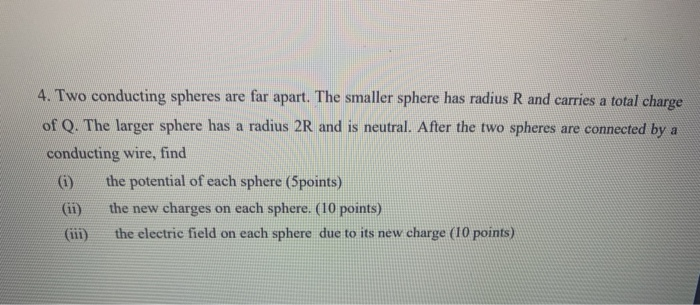4. Two conducting spheres are far apart. The smaller sphere has radius R and carries a total charge of Q. The larger sphere has a radius 2R and is neutral. After the two spheres are connected by a conducting wire, find (1) the potential of each sphere (5points) the new charges on each sphere. (10 points) the electric field on each sphere due to its new charge (10 points)

• Charge is placed on two conducting spheres that are very far apart and connected by a...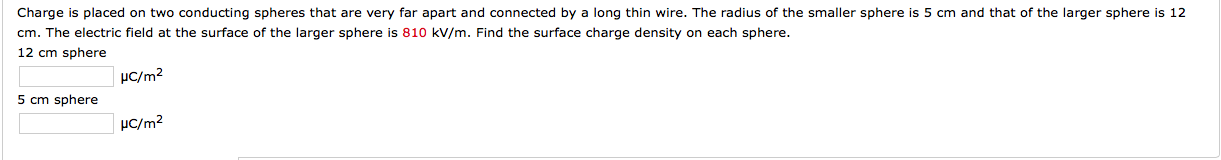Charge is placed on two conducting spheres that are very far apart and connected by a long thin wire. The radius of the smaller sphere is 5 cm and that of the larger sphere is 12 cm. The electric field at the surface of the larger sphere is 810 kV/m. Find the surface charge density on each sphere.

• Charge is placed on two conducting spheres that are very far apart and connected by a...

Charge is placed on two conducting spheres that are very far apart and connected by a long thin wire. The radius of the smaller sphere is 5 cm and that of the larger sphere is 12 cm. The electric field at the surface of the larger sphere is 800 kV/m. Find the surface charge density on each sphere. 12 cm sphere in µC/m2.............................. 5 cm sphere in µC/m2...................................

• Charge is placed on two conducting spheres that are very far apart and connected by a...

Charge is placed on two conducting spheres that are very far apart and connected by a long thin wire. The radius of the smaller sphere is 5 cm and that of the larger sphere is 12 cm. The electric field at the surface of the larger sphere is 490 kV/m. Find the surface charge density on each sphere. 12 cm sphere ? µC/m^2 5 cm sphere ? µC/m^2

• two conducting spheres

Charge is placed on two conducting spheres that are very far apart and connected by a long thin wire. The radius of the smaller sphere is 5 cm and that of the largersphere is 12 cm. The electric field at the surface of the larger sphere is 630 kV/m. Find the surface charge density on each sphere.

• R Q1-Ch23 A conducting solid sphere of radius R with unknown charge Q is at the...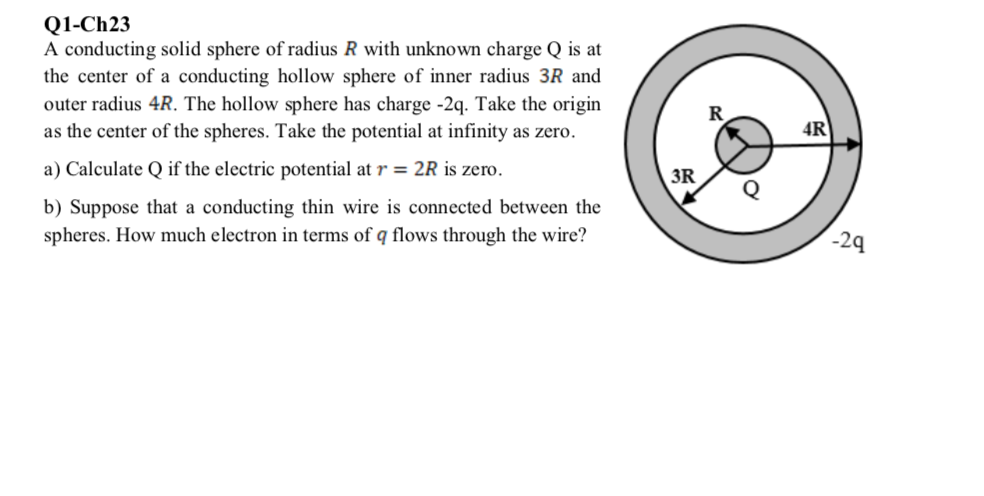R Q1-Ch23 A conducting solid sphere of radius R with unknown charge Q is at the center of a conducting hollow sphere of inner radius 3R and outer radius 4R. The hollow sphere has charge -2q. Take the origin as the center of the spheres. Take the potential at infinity as zero. a) Calculate Q if the electric potential at r = 2R is zero. b) Suppose that a conducting thin wire is connected between the spheres. How much electron...

• Two conducting spheres with radius r1 and r2 and charges q1 and q2, respectively, are connected...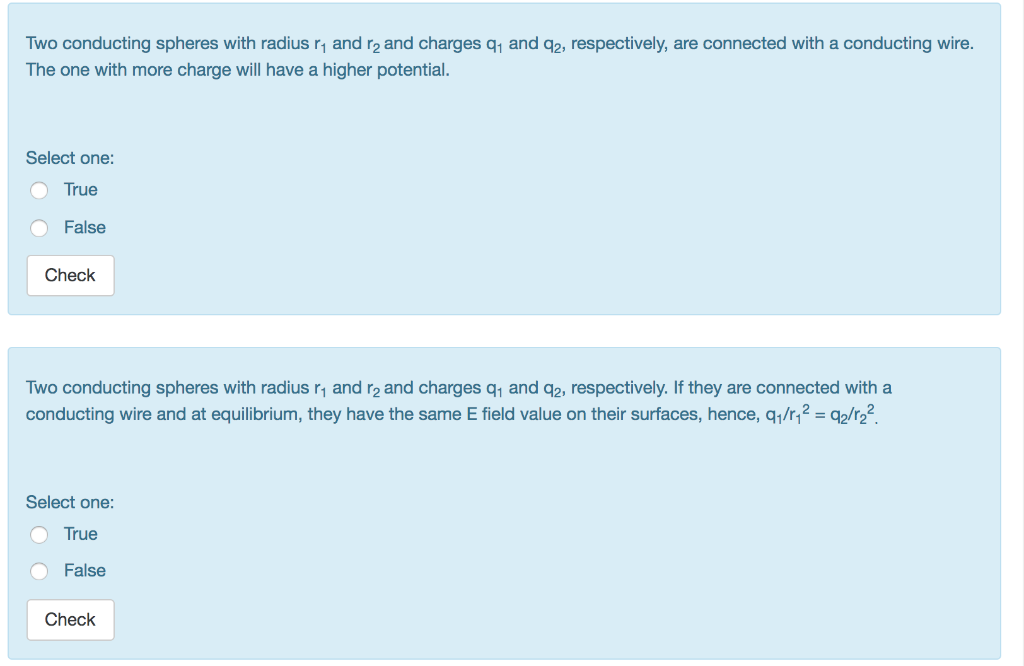Two conducting spheres with radius r1 and r2 and charges q1 and q2, respectively, are connected with a conducting wire. The one with more charge will have a higher potential. Select one: True O False Check Two conducting spheres with radius r1 and r2 and charges q1 and q2, respectively. If they are connected with a conducting wire and at equilibrium, they have the same E field value on their surfaces, hence, q/r,2 2/2 Select one: False Check Two conducting...